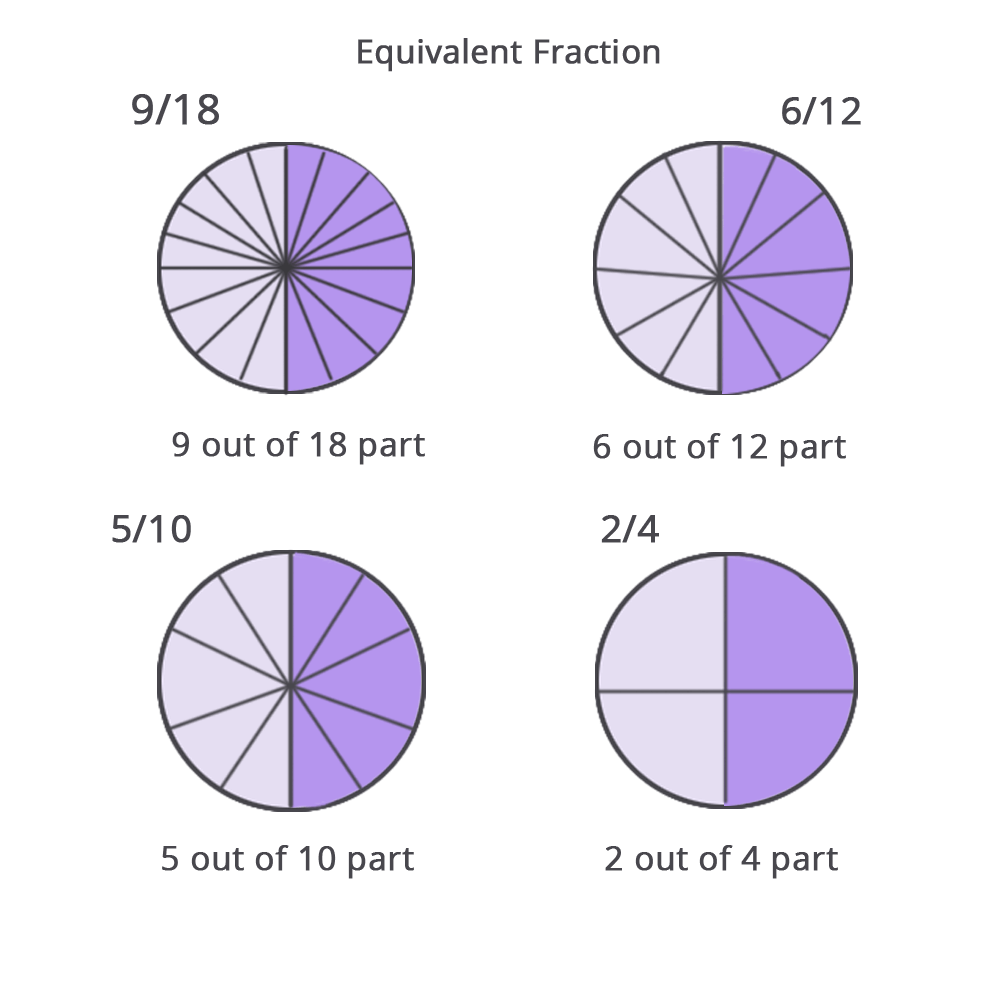• Call Now

1800-102-2727•

# Equivalent Fractions - Examples, How to find, Multiplication, Division and Important Reminders

Equivalent fractions are fractions with the same numerator and denominator but distinct denominators and numerators. 8/24 and 6/18, for example, are comparable fractions since they are both equal to 1/3.

As seen in the example above, the equivalent fractions are at last simplified to the same fraction. Examine the provided lesson to better understand how to locate equivalent fractions as well as determine whether or not the fractions given in the questions are equivalent.

A set of fractions that can be simplified to the same fraction can be claimed to be equivalent. 3/15, 8/40, and 2/10, for example, are comparable fractions of 1/5 that, when simplified, provide the same fraction, 1/5.//Figure 1//
Here are some comparable fractions instances. 9/18, 6/12, 5/10, and 2/4, for example, are equal fractions. Let's look at how their valuations are comparable. Each fraction will be shown as a circle with shaded sections.

## Equivalent Fractions: How to find them?

They are those fractions in which the numerator and denominator are both multiplied or divided by the same value. This is why when these fractions are simplified; they render the same value. Let's look at how we may construct comparable fractions in two different ways:

• Multiplication of both the numerator and denominator by the same number.
• Division of the numerator and denominator by the same number.

## 1. Multiplication of both the numerator and denominator by the same number.

Multiply the numerator and denominator by the same number to get comparable fractions for each given fraction.

## 2. Division of the numerator and denominator by the same number.

The same number can divide numerator and denominator to get comparable fractions for each given fraction. To calculate an equivalent fraction of 36/54, we must first determine their common components. For example, we know that 36 and 54 have a common factor of 2. As a result, dividing the numerator and denominator by two yields an equivalent fraction of 36/54.

## How can you know whether two fractions are equal?

To determine whether the provided fractions are equal, we must simplify them. Simplification can be done to the point where both the numerator and denominator are still whole integers to produce comparable numbers. There are several ways for determining if two fractions are equal. Here are a few examples:

• Getting the denominators to match
• The decimal form of both fractions must be found.
• Method of cross multiplication
• Method using visuals

## Important Reminders

• If the values (decimal/graphical) of two fractions are the same, they are said to be equal.
• To determine a fraction's equivalent fraction, we generally multiply the numerator and denominator by the same amount.
• To test if "any two fractions" are comparable, the "Cross multiplication technique" is employed.
• Another approach for determining if two or more fractions are comparable is making the denominators the same.Talk to our expert
Resend OTP Timer =
By submitting up, I agree to receive all the Whatsapp communication on my registered number and Aakash terms and conditions and privacy policy